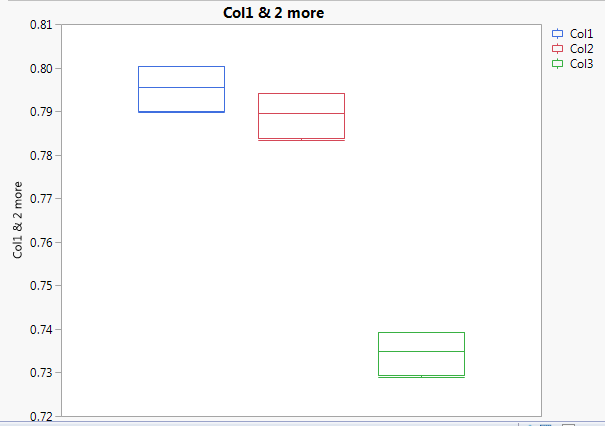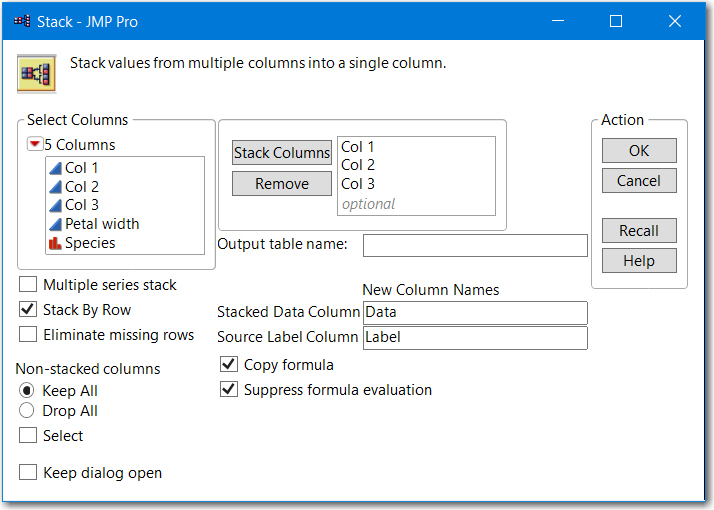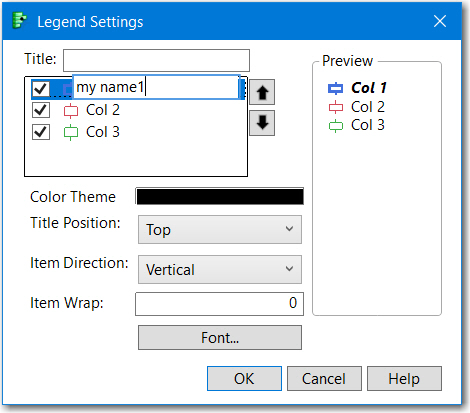Choose Language Hide Translation Bar
Highlighted

## How do I use x-axis label instead of a legend in graph builder box plot?

I would like to have a script that automatically plots several box plots together in jmp.  I am able to come close to what I need, but I don't want to use the legend.  I would like to use the x-axis to label instead.  Below is what I have been able to achieve.  All I need to change is to remove the legend(that's easy) and put the "Col1", "Col2", and Col3" label on the x-axis (can't figure this part out).

The motivation for this change is that I often have a larger number of columns to plot and can't tell which column is which from the color in the legend.  The solution will eventually need to be in JSL, but if I can get it to work manually, it should be simple to extract the script and do it in JSL.1 ACCEPTED SOLUTION

Accepted Solutions
Highlighted

## Re: How do I use x-axis label instead of a legend in graph builder box plot?

The easiest method is to stack your columns first. Then use Data for Y and label for X.

The original plot you created all the points are plotted at zero and the boxplots are offset from the points. There is an X-axis but the range is like -0.5 to 0.5.  You could create some custom labeling to emulate an x-axis but positioning etc, will take a bit of scripting.

By using the stacked columns the points and box plots are aligned, etc.3 REPLIES 3
Highlighted

## Re: How do I use x-axis label instead of a legend in graph builder box plot?

Double click on the legend, then edit the legend names.The JSL will look like this

``````Graph Builder(
Size( 527, 418 ),
Show Control Panel( 0 ),
Variables( Y( :Col 1 ), Y( :Col 2, Position( 1 ) ), Y( :Col 3, Position( 1 ) ) ),
Elements( Box Plot( Y( 1 ), Y( 2 ), Y( 3 ), Legend( 3 ) ) ),
SendToReport(
Dispatch(
{},
"400",
ScaleBox,
{Legend Model(
3,
Level Name( 0, "my name1", Item ID( "Col 1", 1 ) ),
Level Name( 1, "my name 2", Item ID( "Col 2", 1 ) ),
Level Name( 2, "my name 3", Item ID( "Col 3", 1 ) )
)}
)
)
)``````

Highlighted

Highlighted

## Re: How do I use x-axis label instead of a legend in graph builder box plot?

The easiest method is to stack your columns first. Then use Data for Y and label for X.

The original plot you created all the points are plotted at zero and the boxplots are offset from the points. There is an X-axis but the range is like -0.5 to 0.5.  You could create some custom labeling to emulate an x-axis but positioning etc, will take a bit of scripting.

By using the stacked columns the points and box plots are aligned, etc.Article Labels

There are no labels assigned to this post.